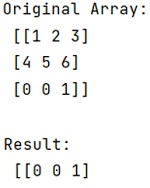# How to Sort a NumPy Array by Column (with Example)?

In this tutorial, we will learn how to sort a NumPy array by column (with example)? By Pranit Sharma Last updated : May 23, 2023

## Problem Statement

Suppose we are given a NumPy array and we need to sort this NumPy array by its nth column.

## Sorting a NumPy Array by Column

To sort a NumPy array by column, the most elegant way of doing it is to use numpy.sort() method where we need to view our array as an array with fields (a structured array). We need to initially define our array with fields otherwise this way is quite ugly.

We can do this in two ways; first, we can sort and return a copy of the array, and second, we can sort it in place.

The advantage of this method is that the order argument is a list of the fields to order the search by.

Let us understand with the help of an example,

## Python Program to Sort a NumPy Array by Column

```# Import numpy
import numpy as np

# Creating a numpy array
arr = np.array([[1,2,3],[4,5,6],[0,0,1]])

# Display Original Array
print("Original Array:\n",arr,"\n")

# Sort it and return a copy
res = np.sort(arr.view('i8,i8,i8'), order=['f1'], axis=0).view(np.int)

# Display Result
print("Result:\n",res,"\n")
```

### Output